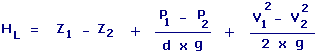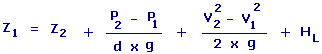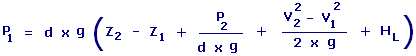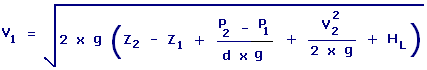Bernoulli Theorem Calculators Enter value, select unit and click on calculate. Result will be displayed.

Calculate Pressure P1
Calculate Velocity V1

 Web www.calculatoredge.comHL = Head Loss d = Density g = Acceleration Of Gravity
 Enter your values: Static Head Z1: Foot Meter Attometer Centimeter Dekameter Decimeter Exameter Femtometer Hectometer Inch Kilometer Megameter Micrometer Mile Millimeter Nanometer Petameter Picometer Yard Static Head Z2: Foot Meter Attometer Centimeter Dekameter Decimeter Exameter Femtometer Hectometer Inch Kilometer Megameter Micrometer Mile Millimeter Nanometer Petameter Picometer Yard Pressure P1: Pound / Foot^2 Pascal Atmosphere Bar Dyne / Centimeter^2 Foot of Water Inch of Mercury Inch of Water Kilonewton / Meter^2 Millibar Newton / Meter^2 Pound / Inch^2 Torr Pressure P2: Pound / Foot^2 Pascal Atmosphere Bar Dyne / Centimeter^2 Foot of Water Inch of Mercury Inch of Water Kilonewton / Meter^2 Millibar Newton / Meter^2 Pound / Inch^2 Torr Velocity V1: Foot / Second Meter / Second Centimeter / Hour Centimeter / Second Foot / Day Inch / Hour Inch / Second Kilometer / Second Knot Meter / Day Mile / Hour Mile / Second Millimeter / Second Yard / Second Velocity V2: Foot / Second Meter / Second Centimeter / Hour Centimeter / Second Foot / Day Inch / Hour Inch / Second Kilometer / Second Knot Meter / Day Mile / Hour Mile / Second Millimeter / Second Yard / Second Density: Pound / Foot^3 Kilogram / Meter^3 Gram / Centimeter^3 Gram / Meter^3 Milligram / Liter Acceleration Of Gravity: Foot / Second^2 Meter / Second^2 Centimeter / Second^2 Inch / Second^2 Kilometer / Second^2 Mile / Second^2 Millimeter / Second^2 Yard / Second^2 Result: Head Loss: Footd = Density g = Acceleration Of Gravity HL = Head Loss
 Enter your values: Static Head Z2: Foot Meter Attometer Centimeter Dekameter Decimeter Exameter Femtometer Hectometer Inch Kilometer Megameter Micrometer Mile Millimeter Nanometer Petameter Picometer Yard Pressure P1: Pound / Foot^2 Pascal Atmosphere Bar Dyne / Centimeter^2 Foot of Water Inch of Mercury Inch of Water Kilonewton / Meter^2 Millibar Newton / Meter^2 Pound / Inch^2 Torr Pressure P2: Pound / Foot^2 Pascal Atmosphere Bar Dyne / Centimeter^2 Foot of Water Inch of Mercury Inch of Water Kilonewton / Meter^2 Millibar Newton / Meter^2 Pound / Inch^2 Torr Velocity V1: Foot / Second Meter / Second Centimeter / Hour Centimeter / Second Foot / Day Inch / Hour Inch / Second Kilometer / Second Knot Meter / Day Mile / Hour Mile / Second Millimeter / Second Yard / Second Velocity V2: Foot / Second Meter / Second Centimeter / Hour Centimeter / Second Foot / Day Inch / Hour Inch / Second Kilometer / Second Knot Meter / Day Mile / Hour Mile / Second Millimeter / Second Yard / Second Density: Pound / Foot^3 Kilogram / Meter^3 Gram / Centimeter^3 Gram / Meter^3 Milligram / Liter Acceleration Of Gravity: Foot / Second^2 Meter / Second^2 Centimeter / Second^2 Inch / Second^2 Kilometer / Second^2 Mile / Second^2 Millimeter / Second^2 Yard / Second^2 Head Loss: Foot Meter Attometer Centimeter Dekameter Decimeter Exameter Femtometer Hectometer Inch Kilometer Megameter Micrometer Mile Millimeter Nanometer Petameter Picometer Yard Result: Static Head Z1: Foot

Calculate Pressure P1d = Density g = Acceleration Of Gravity HL = Head Loss
 Enter your values: Static Head Z1: Foot Meter Attometer Centimeter Dekameter Decimeter Exameter Femtometer Hectometer Inch Kilometer Megameter Micrometer Mile Millimeter Nanometer Petameter Picometer Yard Static Head Z2: Foot Meter Attometer Centimeter Dekameter Decimeter Exameter Femtometer Hectometer Inch Kilometer Megameter Micrometer Mile Millimeter Nanometer Petameter Picometer Yard Pressure P2: Pound / Foot^2 Pascal Atmosphere Bar Dyne / Centimeter^2 Foot of Water Inch of Mercury Inch of Water Kilonewton / Meter^2 Millibar Newton / Meter^2 Pound / Inch^2 Torr Velocity V1: Foot / Second Meter / Second Centimeter / Hour Centimeter / Second Foot / Day Inch / Hour Inch / Second Kilometer / Second Knot Meter / Day Mile / Hour Mile / Second Millimeter / Second Yard / Second Velocity V2: Foot / Second Meter / Second Centimeter / Hour Centimeter / Second Foot / Day Inch / Hour Inch / Second Kilometer / Second Knot Meter / Day Mile / Hour Mile / Second Millimeter / Second Yard / Second Density: Pound / Foot^3 Kilogram / Meter^3 Gram / Centimeter^3 Gram / Meter^3 Milligram / Liter Acceleration Of Gravity: Foot / Second^2 Meter / Second^2 Centimeter / Second^2 Inch / Second^2 Kilometer / Second^2 Mile / Second^2 Millimeter / Second^2 Yard / Second^2 Head Loss: Foot Meter Attometer Centimeter Dekameter Decimeter Exameter Femtometer Hectometer Inch Kilometer Megameter Micrometer Mile Millimeter Nanometer Petameter Picometer Yard Result: Pressure P1: Pound/Foot2

Calculate Velocity V1d = Density g = Acceleration Of Gravity HL = Head Loss
 Enter your values: Static Head Z1: Foot Meter Attometer Centimeter Dekameter Decimeter Exameter Femtometer Hectometer Inch Kilometer Megameter Micrometer Mile Millimeter Nanometer Petameter Picometer Yard Static Head Z2: Foot Meter Attometer Centimeter Dekameter Decimeter Exameter Femtometer Hectometer Inch Kilometer Megameter Micrometer Mile Millimeter Nanometer Petameter Picometer Yard Pressure P1: Pound / Foot^2 Pascal Atmosphere Bar Dyne / Centimeter^2 Foot of Water Inch of Mercury Inch of Water Kilonewton / Meter^2 Millibar Newton / Meter^2 Pound / Inch^2 Torr Pressure P2: Pound / Foot^2 Pascal Atmosphere Bar Dyne / Centimeter^2 Foot of Water Inch of Mercury Inch of Water Kilonewton / Meter^2 Millibar Newton / Meter^2 Pound / Inch^2 Torr Velocity V2: Foot / Second Meter / Second Centimeter / Hour Centimeter / Second Foot / Day Inch / Hour Inch / Second Kilometer / Second Knot Meter / Day Mile / Hour Mile / Second Millimeter / Second Yard / Second Density: Pound / Foot^3 Kilogram / Meter^3 Gram / Centimeter^3 Gram / Meter^3 Milligram / Liter Acceleration Of Gravity: Foot / Second^2 Meter / Second^2 Centimeter / Second^2 Inch / Second^2 Kilometer / Second^2 Mile / Second^2 Millimeter / Second^2 Yard / Second^2 Head Loss: Foot Meter Attometer Centimeter Dekameter Decimeter Exameter Femtometer Hectometer Inch Kilometer Megameter Micrometer Mile Millimeter Nanometer Petameter Picometer Yard Result: Velocity V1: Foot/SecondLINKSDISCLAIMERCONTACT US